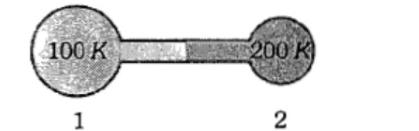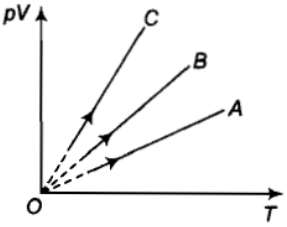The root mean square speed of the molecules of an enclosed gas is V.  What will be the root mean square speed if the pressure is doubled, the temperature remaining the same?

(1) $\frac{v}{2}$      (2) v          (3) 2        (4) 4 v

Concept Questions :-

Types of velocity
High Yielding Test Series + Question Bank - NEET 2020

Difficulty Level:

If the degree of freedom of gas are f, then the ratio of two specific heats ${C}_{P}/{C}_{V}$ is given by:

[MP PET 1995; BHU 1997; MP PMT 2001, 04]

(1) $\frac{2}{f}+1$                   (2) $1-\frac{2}{f}$

(3) $1+\frac{1}{f}$                   (4) $1-\frac{1}{f}$

Concept Questions :-

Specific heat
High Yielding Test Series + Question Bank - NEET 2020

Difficulty Level:

The equation $\left(p+\frac{a}{{v}^{2}}\right)\left(v-b\right)=RT$ is known as:

(1) Perfect gas equation

(2) Joule Thomson's equation

(3) Vander Waal's equation

(4) Maxwell's equation

Concept Questions :-

Ideal gas
High Yielding Test Series + Question Bank - NEET 2020

Difficulty Level:

The temperature of an ideal gas is increased from $27°$ to $927°C$. The r.m.s. speed of its molecules becomes-

(1) twice            (2) half            (3) four times          (4) one fourth

Concept Questions :-

Types of velocity
High Yielding Test Series + Question Bank - NEET 2020

Difficulty Level:

An ideal gas is filled in a vessel, then

(1) If it is placed inside a moving train, its temperature increases

(2) Its centre of mass moves randomly

(3) Its temperature remains constant in a moving car

(4) None of these

Concept Questions :-

Kinetic energy of gas
High Yielding Test Series + Question Bank - NEET 2020

Difficulty Level:

If the molecular weight of two gases are , then at a temperature the ratio of root mean square velocity  will be: [MP PMT 1996; AMU (Engg.) 2000; CPMT 2000; DPMT 2001]

(1) $\sqrt{\frac{{M}_{1}}{{M}_{2}}}$                          (2) $\sqrt{\frac{{M}_{2}}{{M}_{1}}}$

(3) $\sqrt{\frac{{M}_{1}+{M}_{2}}{{M}_{1}-{M}_{2}}}$                  (4) $\sqrt{\frac{{M}_{1}-{M}_{2}}{{M}_{1}+{M}_{2}}}$

Concept Questions :-

Types of velocity
High Yielding Test Series + Question Bank - NEET 2020

Difficulty Level:

An ideal gas is found to obey an additional law $V{P}^{2}$= constant. The gas is initially at temperature T and volume V. When it expands to a volume 2 V, the temperature becomes:

(1) T $\sqrt{2}$         (2) 2T         (3) 2T$\sqrt{2}$           (4) 4T

Concept Questions :-

Ideal gas
High Yielding Test Series + Question Bank - NEET 2020

Difficulty Level:

According to the kinetic theory of gases, at absolute temperature: [AIIM 1998; UPSEAT 2000]

(1) Water freezes                             (2) Liquid helium freezes

(3) Molecular motion stops               (3) Liquid hydrogen freezes

Concept Questions :-

Types of velocity
High Yielding Test Series + Question Bank - NEET 2020

Difficulty Level:

The figure shows two flasks connected to each other. The volume of the flask 1 is twice that of flask 2.  The system is filled with an ideal gas at temperature of 100 K and 200 K respectively.  If the mass of the gas in 1 be m then what is the mass of the gas in flask 2 [CBSE PMT 2000](1) m              (2) m/2       (3) m/4        (4) m/8

Concept Questions :-

Ideal gas
High Yielding Test Series + Question Bank - NEET 2020

Difficulty Level:

PV versus T graph of equal masses of  is shown in the figure. Choose the correct alternative.1.  A corresponds to H2, B to He and C to O2

2.  A corresponds to He, B to H2 and C to O2

3.  A corresponds to He, B to O2 and C to H2

4.  A corresponds to O2, B to He  and C to H2

Concept Questions :-

Ideal gas